# Testing your Java with Groovy, Spock, JUnit5, Jacoco, Jqwik and Pitest

Author: Paul King
Published: 2022-07-15 08:26AMThis blog post covers a common scenario seen in the Groovy community which is projects which use Java for their production code and Groovy for their tests. This can be a low risk way for Java shops to try out and become more familiar with Groovy. We’ll write our initial tests using the Spock testing framework, and we’ll use JUnit5 later with our jqwik tests. You can usually use your favorite Java testing libraries if you switch to Groovy.

## The system under test

For illustrative purposes, we will test a Java mathematics utility function `sumBiggestPair`. Given three numbers, it finds the two biggest and then adds them up. An initial stab at the code for this might look something like this:

``````public class MathUtil {                       // Java

public static int sumBiggestPair(int a, int b, int c) {
int op1 = a;
int op2 = b;
if (c > a) {
op1 = c;
} else if (c > b) {
op2 = c;
}
return op1 + op2;
}

private MathUtil(){}
}``````

## Testing with Spock

An initial test could look like this:

``````class MathUtilSpec extends Specification {
def "sum of two biggest numbers"() {
expect:
MathUtil.sumBiggestPair(2, 5, 3) == 8
}
}``````

When we run this test, all tests pass:But if we look at the coverage report, generated with Jacoco, we see that our test hasn’t covered all lines of code: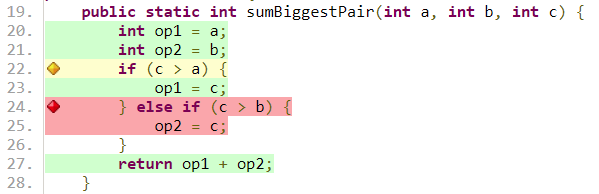We’ll swap to use Spock’s data-driven feature and include an additional testcase:

``````def "sum of two biggest numbers"(int a, int b, int c, int d) {
expect:
MathUtil.sumBiggestPair(a, b, c) == d

where:
a | b | c | d
2 | 5 | 3 | 8
5 | 2 | 3 | 8
}``````

We can check our coverage again: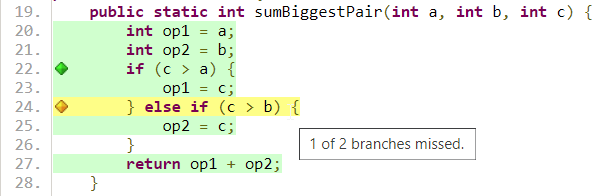That is a little better. We now have 100% line coverage but not 100% branch coverage. Let’s add one more testcase:

``````def "sum of two biggest numbers"(int a, int b, int c, int d) {
expect:
MathUtil.sumBiggestPair(a, b, c) == d

where:
a | b | c | d
2 | 5 | 3 | 8
5 | 2 | 3 | 8
5 | 4 | 1 | 9
}``````

And now we can see that we have reached 100% line coverage and 100% branch coverage: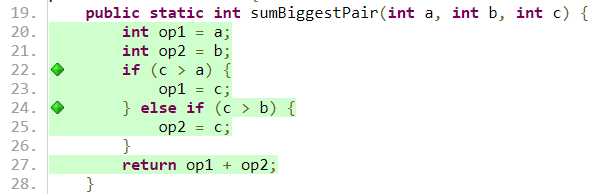At this point, we might be very confident in our code and ready to ship it to production. Before we do, we’ll add one more testcase:

``````def "sum of two biggest numbers"(int a, int b, int c, int d) {
expect:
MathUtil.sumBiggestPair(a, b, c) == d

where:
a | b | c | d
2 | 5 | 3 | 8
5 | 2 | 3 | 8
5 | 4 | 1 | 9
3 | 2 | 6 | 9
}``````

When we re-run our tests, we discover that the last testcase failed!: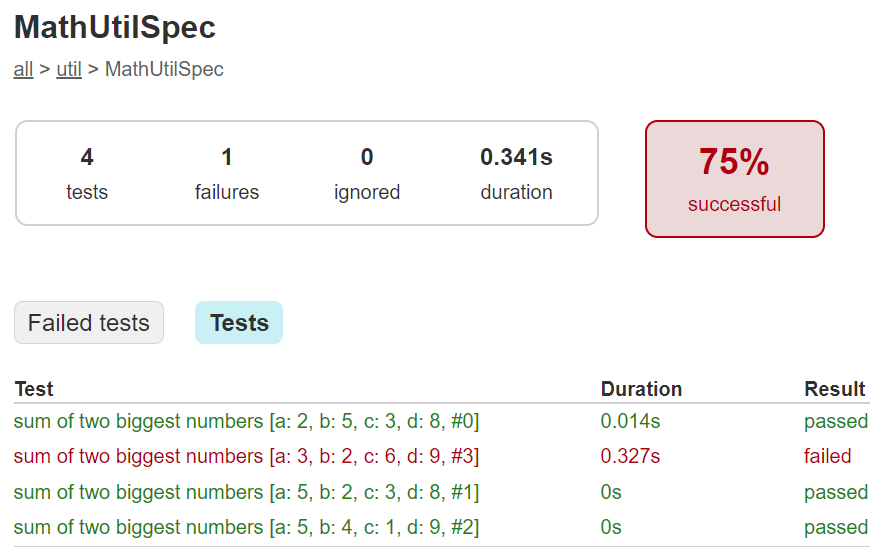And examining the testcase, we can indeed see that there is a flaw in our algorithm. Basically, having the `else` logic doesn’t cater for when `c` is greater than both `a` and `b`!We succumbed to faulty expectations of what 100% coverage would give us.The good news is that we can fix this. Here is an updated algorithm:

``````public static int sumBiggestPair(int a, int b, int c) {                // Java
int op1 = a;
int op2 = b;
if (c > Math.min(a, b)) {
op1 = c;
op2 = Math.max(a, b);
}
return op1 + op2;
}``````

With this new algorithm, all 4 testcases now pass, and we again have 100% line and branch coverage.

```> Task :SumBiggestPairPitest:test
✔ Test sum of two biggest numbers [Tests: 4/4/0/0] [Time: 0.317 s]
✔ Test util.MathUtilSpec [Tests: 4/4/0/0] [Time: 0.320 s]
✔ Test Gradle Test Run :SumBiggestPairPitest:test [Tests: 4/4/0/0]```

But haven’t we been here before? How can we be sure there isn’t some additional test cases that might reveal another flaw in our algorithm? We could keep writing lots more testcases, but we’ll look at two other techniques that can help.

## Mutation testing with Pitest

An interesting but not widely used technique is mutation testing. It probably deserves to be more widely used. It can test the quality of a testsuite but has the drawback of sometimes being quite resource intensive. It modifies (mutates) production code and re-runs your testsuite. If your test suite still passes with modified code, it possibly indicates that your testsuite is lacking sufficient coverage. Earlier, we had an algorithm with a flaw and our testsuite didn’t initially pick it up. You can think of mutation testing as adding a deliberate flaw and seeing whether your testsuite is good enough to detect that flaw.

If you’re a fan of test-driven development (TDD), it espouses a rule that not a single line of production code should be added unless a failing test forces that line to be added. A corollary is that if you change a single line of production code in any meaningful way, that some test should fail.

So, let’s have a look at what mutation testing says about our initial flawed algorithm. We’ll use Pitest (also known as PIT). We’ll go back to our initial algorithm and the point where we erroneously thought we had 100% coverage. When we run Pitest, we get the following result: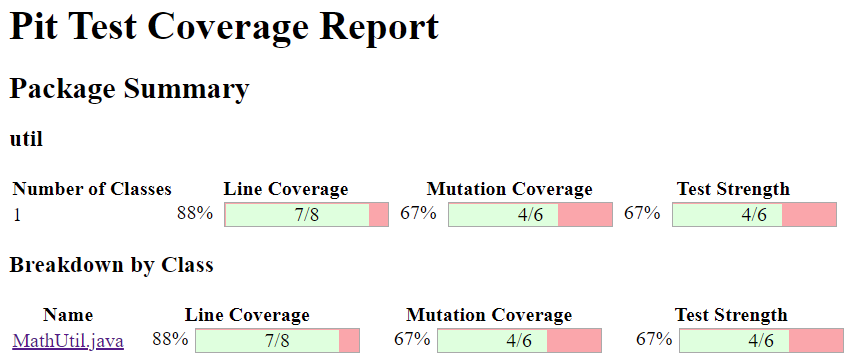And looking at the code we see: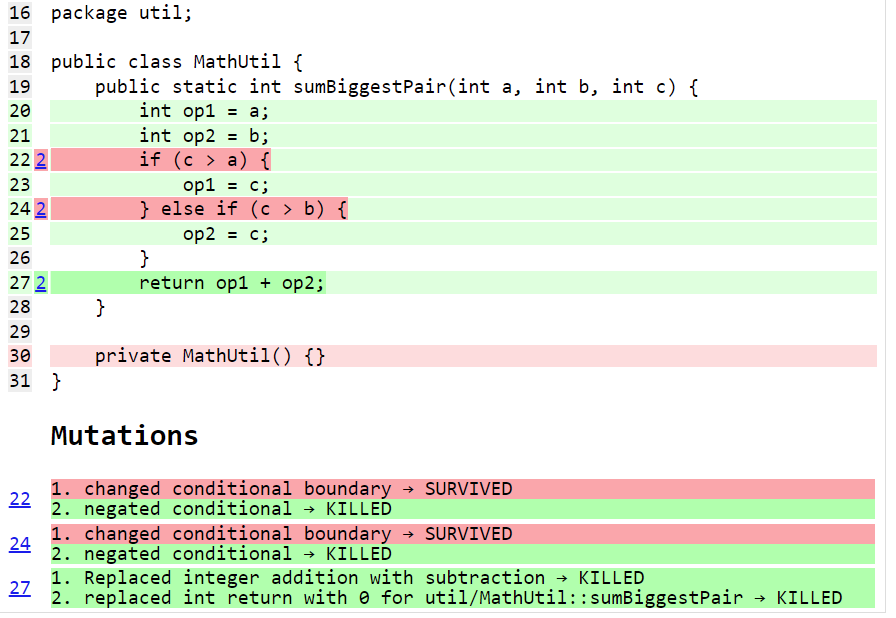With output including some statistics:

```======================================================================
- Statistics
======================================================================
>> Line Coverage: 7/8 (88%)
>> Generated 6 mutations Killed 4 (67%)
>> Mutations with no coverage 0. Test strength 67%
>> Ran 26 tests (4.33 tests per mutation)```

What is this telling us? Pitest mutated our code in ways that you might expect to break it but our testsuite passed (survived) in a couple of instances. That means one of two things. Either, there are multiple valid implementations of our algorithm and Pitest found one of those equivalent solutions, or our testsuite is lacking some key testcases. In our case, we know that the testsuite was insufficient.

Let’s run it again but this time with all of our tests and the corrected algorithm.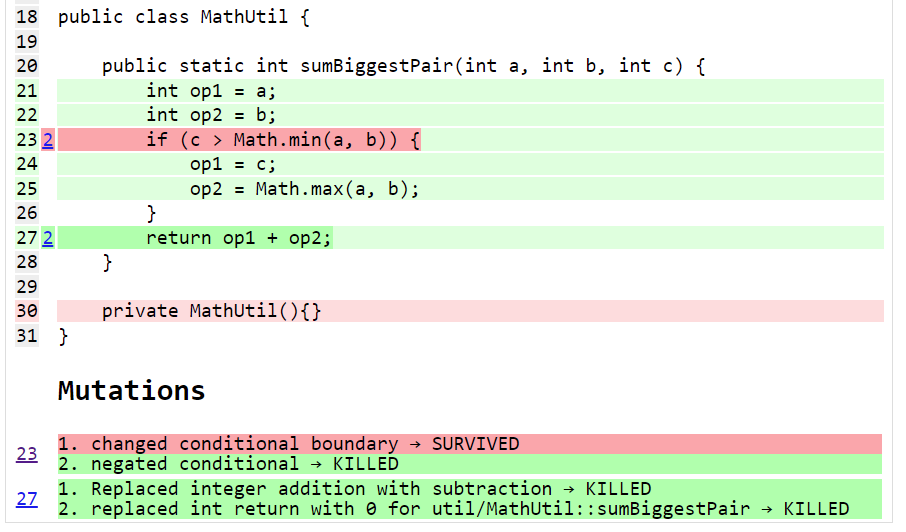The output when running the test has also changed slightly:

```======================================================================
- Statistics
======================================================================
>> Line Coverage: 6/7 (86%)
>> Generated 4 mutations Killed 3 (75%)
>> Mutations with no coverage 0. Test strength 75%
>> Ran 25 tests (6.25 tests per mutation)```

Our warnings from Pitest have reduced but not gone completely away and our test strength has gone up but is still not 100%. It does mean that we are in better shape than before. But should we be concerned?

It turns out in this case, we don’t need to worry (too much). As an example, an equally valid algorithm for our function under test would be to replace the conditional with `c >= Math.min(a, b)`. Note the greater-than-equals operator rather than just greater-than. For this algorithm, a different path would be taken for the case when `c` equals `a` or `b`, but the end result would be the same. So, that would be an inconsequential or equivalent mutation. In such a case, there may be no additional testcase that we can write to keep Pitest happy. We have to be aware of this possible outcome when using this technique.

Finally, let’s look at our build file that ran Spock, Jacoco and Pitest:

``````plugins {
id 'info.solidsoft.pitest' version '1.7.4'
}
apply plugin: 'groovy'

repositories {
mavenCentral()
}

dependencies {
implementation "org.apache.groovy:groovy-test-junit5:4.0.3"
testImplementation("org.spockframework:spock-core:2.2-M3-groovy-4.0") {
transitive = false
}
}

pitest {
junit5PluginVersion = '1.0.0'
pitestVersion = '1.9.2'
timestampedReports = false
targetClasses = ['util.*']
}

useJUnitPlatform()
}``````

The astute reader might note some subtle hints which show that the latest Spock versions run on top of the JUnit 5 platform.

## Using Property-based Testing

Property-based testing is another technology which probably deserves much more attention. Here we’ll use jqwik which runs on top of JUnit5, but you might also like to consider Genesis which provides random generators and especially targets Spock.

Earlier, we looked at writing more tests to make our coverage stronger. Property-based testing can often lead to writing less tests. Instead, we generate many random tests automatically and see whether certain properties hold.

Previously, we fed in the inputs and the expected output. For property-based testing, the inputs are typically randomly-generated values, we don’t know the output. So, instead of testing directly against some known output, we’ll just check various properties of the answer.

As an example, here is a test we could use:

``````@Property
void "result should be bigger than any individual and smaller than sum of all"(
@ForAll @IntRange(min = 0, max = 1000) Integer a,
@ForAll @IntRange(min = 0, max = 1000) Integer b,
@ForAll @IntRange(min = 0, max = 1000) Integer c) {
def result = sumBiggestPair(a, b, c)
assert [a, b, c].every { individual -> result >= individual }
assert result <= a + b + c
}``````

The `@ForAll` annotations indicate places where jqwik will insert random values. The `@IntRange` annotation indicates that we want the random values to be contained between 0 and 1000.

Here we are checking that (at least for small positive numbers) adding the two biggest numbers should be greater than or equal to any individual number and should be less than or equal to adding all three of the numbers. These are necessary but insufficient properties to ensure our system works.

When we run this we see the following output in the logs:

```                              |--------------------jqwik--------------------
tries = 1000                  | # of calls to property
checks = 1000                 | # of not rejected calls
generation = RANDOMIZED       | parameters are randomly generated
after-failure = PREVIOUS_SEED | use the previous seed
when-fixed-seed = ALLOW       | fixing the random seed is allowed
edge-cases#mode = MIXIN       | edge cases are mixed in
edge-cases#total = 125        | # of all combined edge cases
edge-cases#tried = 117        | # of edge cases tried in current run
seed = -311315135281003183    | random seed to reproduce generated values```

So, we wrote 1 test and 1000 testcases were executed. The number of tests run is configurable. We won’t go into the details here. This looks great at first glance. It turns out however, that this particular property is not very discriminating in terms of the bugs it can find. This test passes for both our original flawed algorithm as well as the fixed one. Let’s try a different property:

``````@Property
void "sum of any pair should not be greater than result"(
@ForAll @IntRange(min = 0, max = 1000) Integer a,
@ForAll @IntRange(min = 0, max = 1000) Integer b,
@ForAll @IntRange(min = 0, max = 1000) Integer c) {
def result = sumBiggestPair(a, b, c)
assert [a + b, b + c, c + a].every { sumOfPair -> result >= sumOfPair }
}``````

If we calculate the biggest pair, then surely it must be greater than or equal to any arbitrary pair. Trying this on our flawed algorithm gives:

```org.codehaus.groovy.runtime.powerassert.PowerAssertionError:
assert [a + b, b + c, c + a].every { sumOfPair -> result >= sumOfPair }
| | |  | | |  | | |  |
1 1 0  0 2 2  2 3 1  false
|--------------------jqwik--------------------
tries = 12                    | # of calls to property
checks = 12                   | # of not rejected calls
generation = RANDOMIZED       | parameters are randomly generated
after-failure = PREVIOUS_SEED | use the previous seed
when-fixed-seed = ALLOW       | fixing the random seed is allowed
edge-cases#mode = MIXIN       | edge cases are mixed in
edge-cases#total = 125        | # of all combined edge cases
edge-cases#tried = 2          | # of edge cases tried in current run
seed = 4830696361996686755    | random seed to reproduce generated values

Shrunk Sample (6 steps)
-----------------------
arg0: 1
arg1: 0
arg2: 2

Original Sample
---------------
arg0: 247
arg1: 32
arg2: 267

Original Error
--------------
org.codehaus.groovy.runtime.powerassert.PowerAssertionError:
assert [a + b, b + c, c + a].every { sumOfPair -> result >= sumOfPair }
| | |  | | |  | | |  |
| | 32 32| 267| | |  false
| 279    299  | | 247
247           | 514
267```

Not only did it find a case which highlighted the flaw, but it shrunk it down to a very simple example. On our fixed algorithm, the 1000 tests pass!

The previous property can be refactored a little to not only calculate all three pairs but then find the maximum of those. This simplifies the condition somewhat:

``````@Property
void "result should be the same as alternative oracle implementation"(
@ForAll @IntRange(min = 0, max = 1000) Integer a,
@ForAll @IntRange(min = 0, max = 1000) Integer b,
@ForAll @IntRange(min = 0, max = 1000) Integer c) {
assert sumBiggestPair(a, b, c) == [a+b, a+c, b+c].max()
}``````

This approach, where an alternative implementation is used, is known as a test oracle. The alternative implementation might be less efficient, so not ideal for production code, but fine for testing. When revamping or replacing some software, the oracle might be the existing system. When run on our fixed algorithm, we again have 1000 testcases passing.

Let’s go one step further and remove our `@IntRange` boundaries on the Integers:

``````@Property
void "result should be the same as alternative oracle implementation"(@ForAll Integer a, @ForAll Integer b, @ForAll Integer c) {
assert sumBiggestPair(a, b, c) == [a+b, a+c, b+c].max()
}``````

When we run the test now, we might be surprised:

```  org.codehaus.groovy.runtime.powerassert.PowerAssertionError:
assert sumBiggestPair(a, b, c) == [a+b, a+c, b+c].max()
|              |  |  |  |   |||  |||  |||  |
-2147483648    0  1  |  |   0|1  0||  1||  2147483647
|  |    1    ||   |2147483647
|  false     ||   -2147483648
2147483647   |2147483647
2147483647
Shrunk Sample (13 steps)
------------------------
arg0: 0
arg1: 1
arg2: 2147483647```

It fails! Is this another bug in our algorithm? Possibly? But it could equally be a bug in our property test. Further investigation is warranted.

It turns out that our algorithm suffers from Integer overflow when trying to add `1` to `Integer.MAX_VALUE`. Our test partially suffers from the same problem but when we call `max()`, the negative value will be discarded. There is no always correct answer as to what should happen in this scenario. We go back to the customer and check the real requirement. In this case, let’s assume the customer was happy for the overflow to occur - since that is what would happen if performing the operation long-hand in Java. With that knowledge we should fix our test to at least pass correctly when overflow occurs.

We have a number of options to fix this. We already saw previously we can use `@IntRange`. This is one way to "avoid" the problem and we have a few similar approaches which do the same. We could use a more confined data type, e.g. `Short`:

``````@Property
void checkShort(@ForAll Short a, @ForAll Short b, @ForAll Short c) {
assert sumBiggestPair(a, b, c) == [a+b, a+c, b+c].max()
}``````

Or we could use a customised provider method:

``````@Property
void checkIntegerConstrainedProvider(@ForAll('halfMax') Integer a,
@ForAll('halfMax') Integer b,
@ForAll('halfMax') Integer c) {
assert sumBiggestPair(a, b, c) == [a+b, a+c, b+c].max()
}

@Provide
Arbitrary<Integer> halfMax() {
int halfMax = Integer.MAX_VALUE >> 1
return Arbitraries.integers().between(-halfMax, halfMax)
}``````

But rather than avoiding the problem, we could change our test so that it allowed for the possibility of overflow within `sumBiggestPair` but didn’t compound the problem with its own overflow. E.g. we could use Long’s to do our calculations within our test:

``````@Property
void checkIntegerWithLongCalculations(@ForAll Integer a, @ForAll Integer b, @ForAll Integer c) {
def (al, bl, cl) = [a, b, c]*.toLong()
assert sumBiggestPair(a, b, c) == [al+bl, al+cl, bl+cl].max().toInteger()
}``````

Finally, let’s again look at our Gradle build file:

``````apply plugin: 'groovy'

repositories {
mavenCentral()
}

dependencies {
testImplementation project(':SumBiggestPair')
testImplementation "org.apache.groovy:groovy-test-junit5:4.0.3"
testImplementation "net.jqwik:jqwik:1.6.5"
}

test {
useJUnitPlatform {
includeEngines 'jqwik'
}
}``````